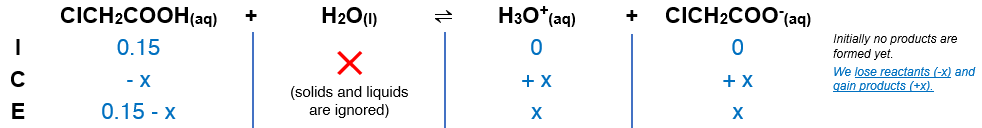# Problem: A 0.15 M solution of chloroacetic acid has a pH of 1.86. What is the value of Ka for this acid?

###### FREE Expert Solution

We are being asked to calculate the Ka for a 0.15 M chloroacetic acid (ClCH2COOH).

Step 1. Equilibrium reaction:        ClCH2COOH(aq) + H2O(l)   H3O+(aq) + ClCH2COO-(aq)$\overline{){{\mathbf{K}}}_{{\mathbf{a}}}{\mathbf{=}}\frac{\mathbf{products}}{\mathbf{reactants}}{\mathbf{=}}\frac{\mathbf{\left[}{\mathbf{H}}_{\mathbf{3}}{\mathbf{O}}^{\mathbf{+}}\mathbf{\right]}\mathbf{\left[}{\mathbf{ClCH}}_{\mathbf{2}}{\mathbf{COO}}^{\mathbf{-}}\mathbf{\right]}}{\left[{\mathrm{ClCH}}_{2}\mathrm{COOH}\right]}}$

${\mathbf{K}}_{\mathbf{a}}\mathbf{=}\frac{\left(x\right)\left(x\right)}{\mathbf{0}\mathbf{.}\mathbf{15}\mathbf{-}\mathbf{x}}\phantom{\rule{0ex}{0ex}}\overline{){{\mathbf{K}}}_{{\mathbf{a}}}{\mathbf{=}}\frac{{\mathbf{x}}^{\mathbf{2}}}{\mathbf{0}\mathbf{.}\mathbf{15}\mathbf{-}\mathbf{x}}}$

86% (72 ratings)###### Problem Details

A 0.15 M solution of chloroacetic acid has a pH of 1.86. What is the value of Ka for this acid?